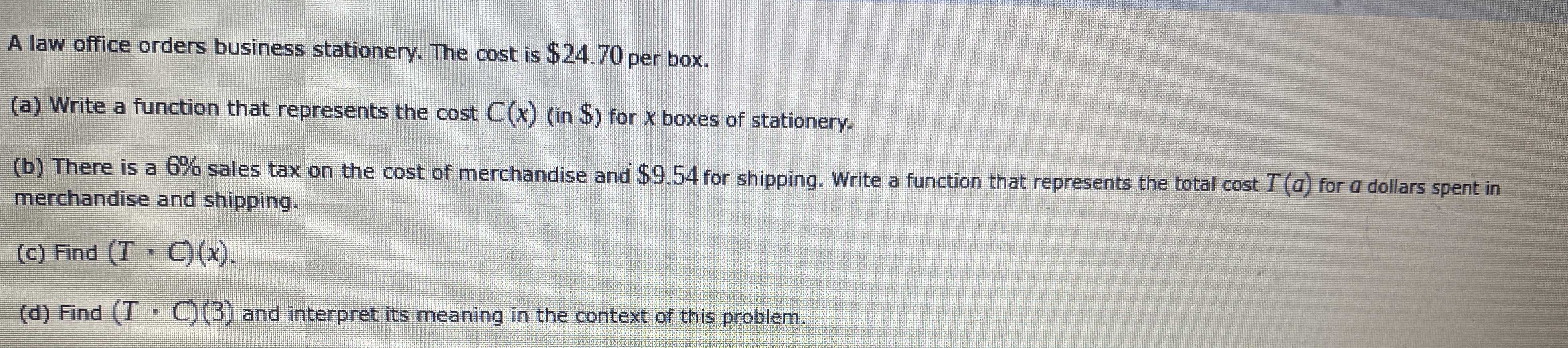### ¿Todavía tienes preguntas de matemáticas?

Pregunte a nuestros tutores expertos
Algebra
PreguntaA law office orders business stationery. The cost is $$\ 24.70$$ per box. (a) Write a function that represents the cost $$C ( x )$$ (in $$\$$ ) for $$x$$ boxes of stationery. (b) There is a $$6 \%$$ sales tax on the cost of merchandise and $$\ 9.54$$ for shipping. Write a function that represents the total cost $$T ( a )$$ for $$a$$ dollars spent in merchandise and shipping. (c) Find $$( T \cdot C ) ( x )$$ . (d) Find $$( T \cdot C ) ( 3 )$$ and interpret its meaning in the context of this problem.

T*C(x)=646.6954x$$^{2}$$+235.638x